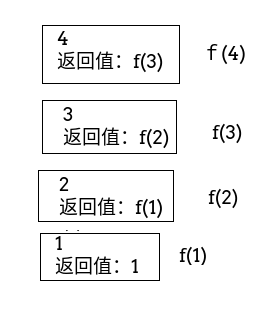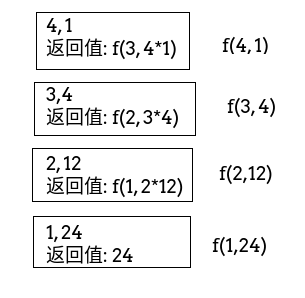# 递归函数两种方式的区别

## 概述

function f($n){ if($n <= 1) return 1;
return $n * f($n-1);
}

function f($n,$result){
if($n <= 1) return$result;
return f($n-1,$n*\$result);
}

## 分析f(1)=1
f(2)=2*f(1)=2*1=2
f(3)=3*f(2)=3*2=6
f(4)=4*f(3)=4*6=24

f(4, 1)=f(3, 4*1)=f(2, 3*4)=f(1, 2*12)=240 评论

0